﻿ How to Insert Equation in Word - TechCult

# How to Insert Equation in Word

Microsoft Equation Editor was available in earlier versions of MS Word. However, developers discontinued and replaced the feature with a new equation editor for all versions released after January 2018. So, if you also struggle with how to insert equation in Word online, this article is for you. We have explained everything in great detail including how to use Microsoft equation editor shortcuts.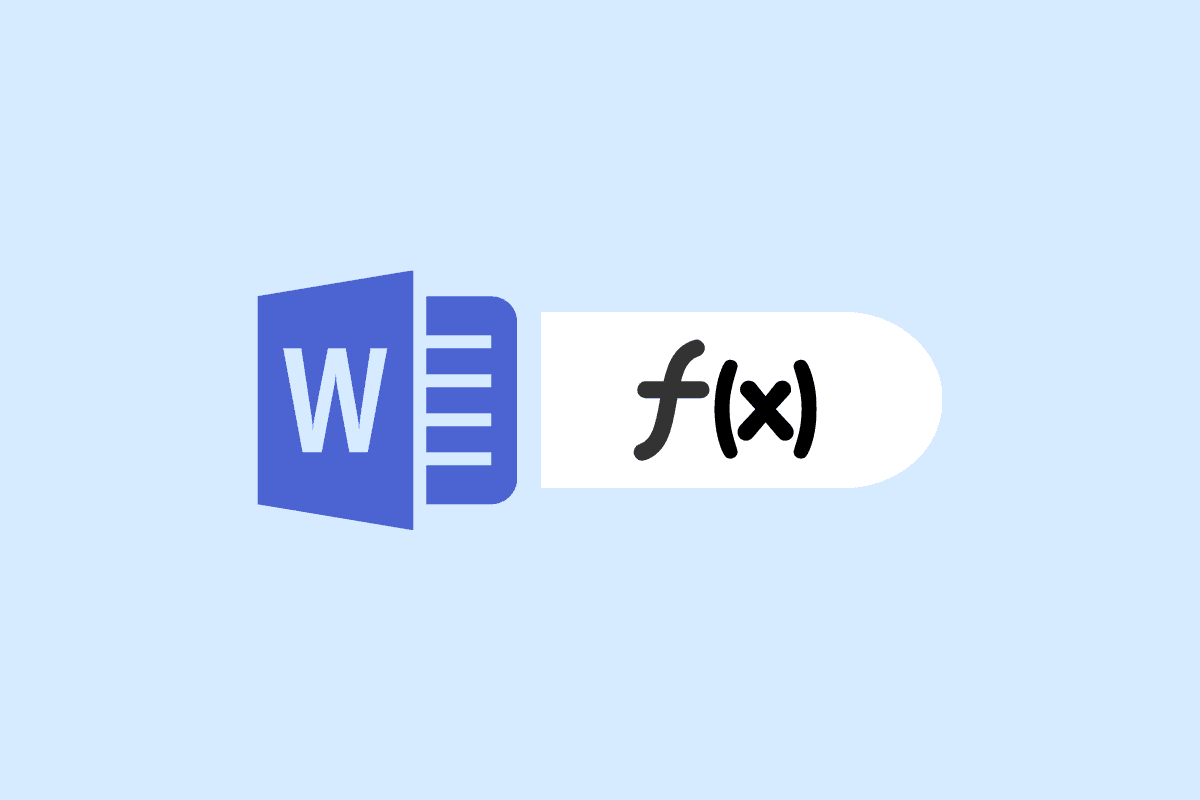## How to Insert Equation in Word

Using the equation editor in MS Word is no big task. Nonetheless, it still troubles netizens as they find it complex. This is a pretty helpful feature that aids the user in inserting complex symbols, structures, and equations like a pro. Continue reading this article to understand how it works.

### What is Microsoft Equation Editor?

Microsoft Equation Editor is an in-built feature in MS Word that can be used to insert equations in Word. You do not have to download Microsoft equation editor as MS Word comes pre-equipped with the feature.

• It consists of preset equation templates that can be edited and used accordingly. The top row of the toolbar consists of more than 150 symbols and the bottom row contains presets of fractions, radicals, summations, integrals, etc.
• It enables users to build complex equations using the toolbar which consists of a wide range of symbols.

### How to Insert Equation in Word Online

Now that you know well about the equation editor in Word, let us move forward with how to do it.

1. Browse online MS Word on your Windows laptop.

2. Open the Word document in which you wish to insert the equation.

3. Next, click on the Insert tab from the set of toolbars on the top.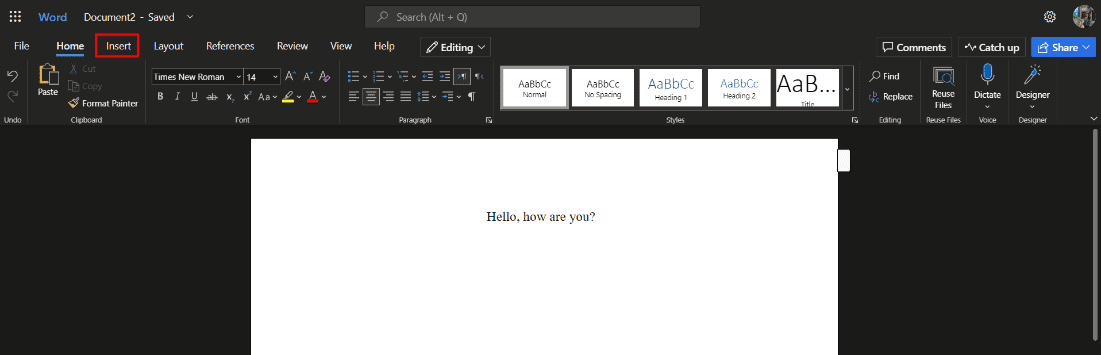4. Click on the Equation option next to Symbol and choose the required symbols or structures for the document.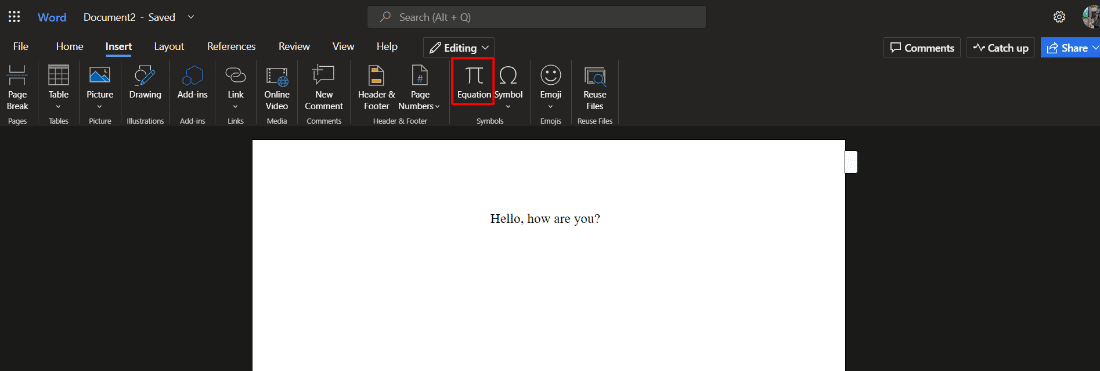### How to Use Microsoft Equation Editor in Word Online?

To know how to use Microsoft Equation Editor, all you have to do is follow the below-mentioned steps:

1. Browse online MS Word on your Windows laptop.

2. Open the document where you need the equation.

3. Next, click on the Insert and then on Equation.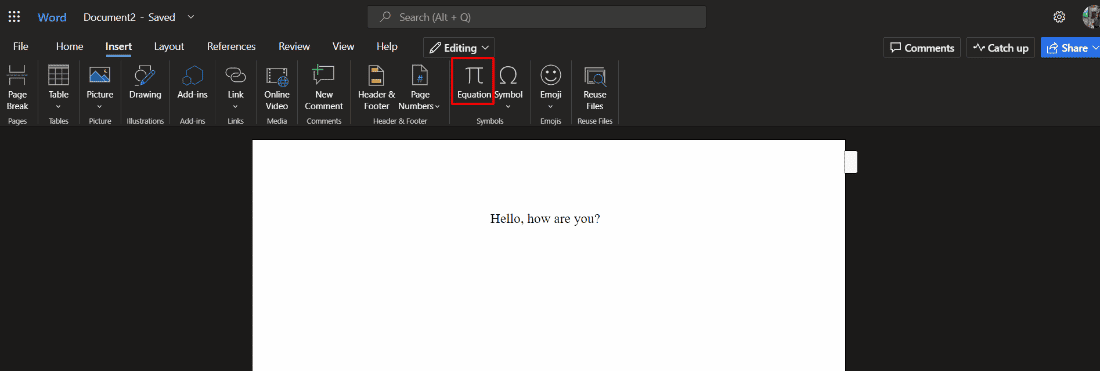4. Enter your Microsoft equation editor shortcuts in the space provided below Equation Tools.5. Finally, click on Insert on page option.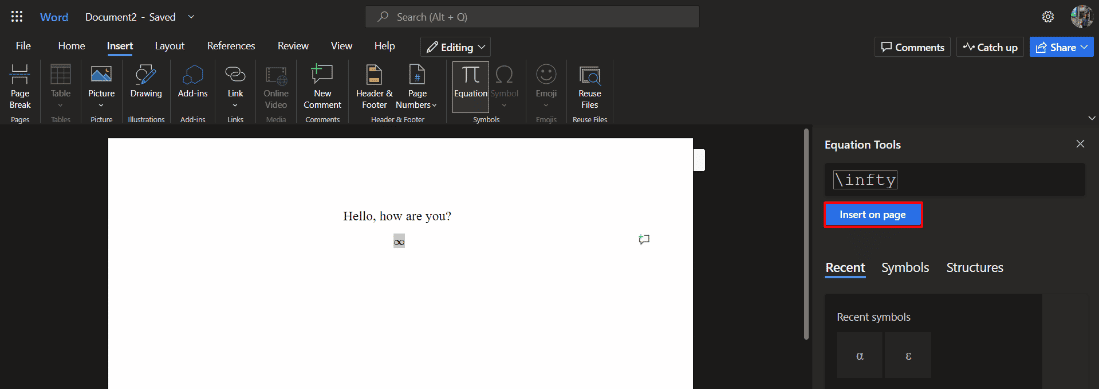### How to Insert Equation in Word 2007?

Below we have mentioned the different methods to understand how to do it offline in MS Office app, specifically focussing on Word 2007 onwards.

#### Method 1: Insert Preset Equations

Follow the below-mentioned steps to insert equation in Word by using the in-built presets:

1. Click on the Insert option in the menu bar.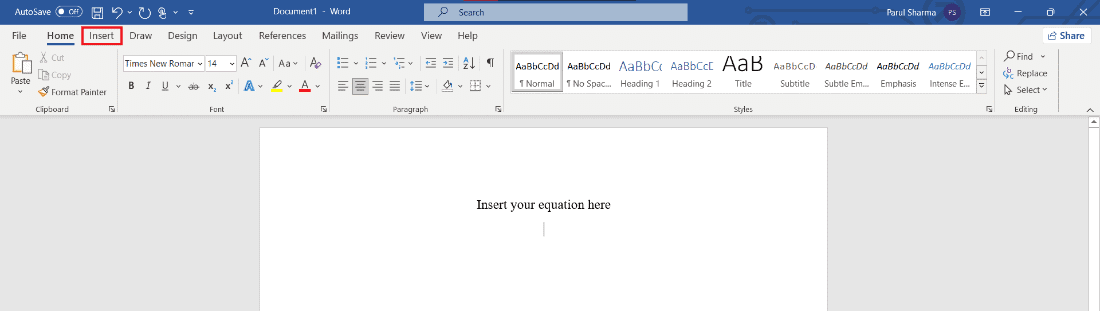2. Then, click on Equation on the toolbar.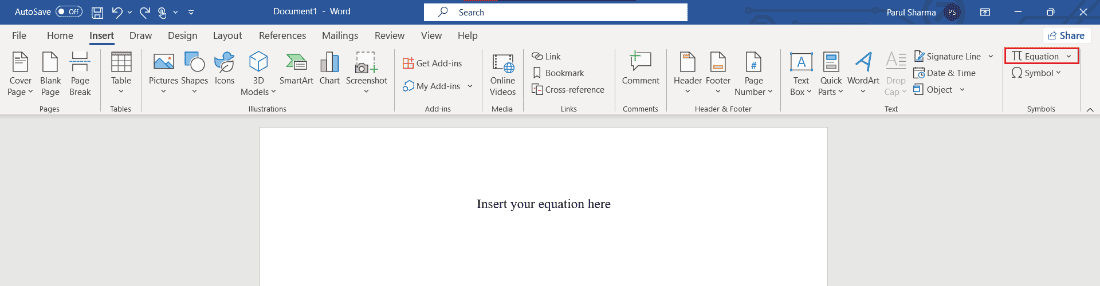3. Scroll down and click on the required equation.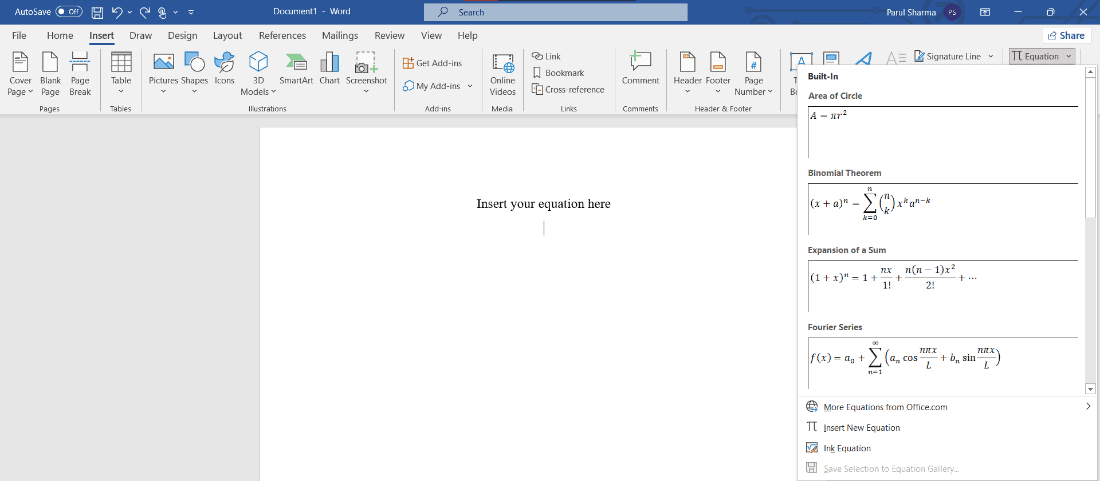The selected equation will appear in your working area and you can edit it accordingly.

#### Method 2: Insert Equation Manually

Follow the steps listed below to create an equation manually on MS Word:

1. Click on the Insert option in the menu bar.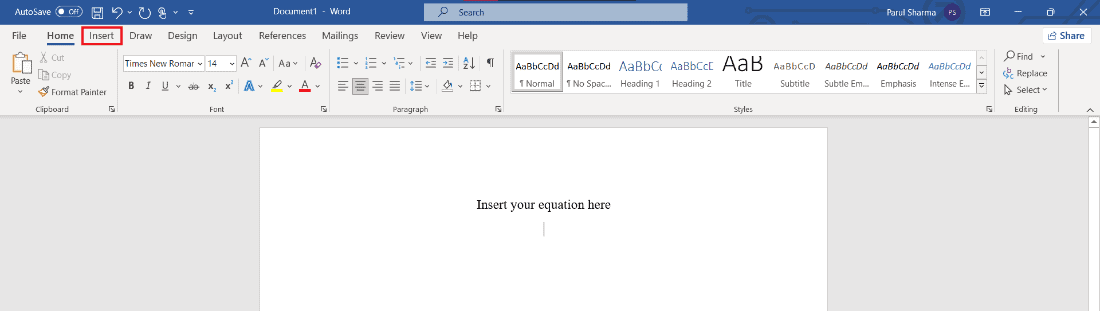2. Then, click on the Equation icon on the toolbar.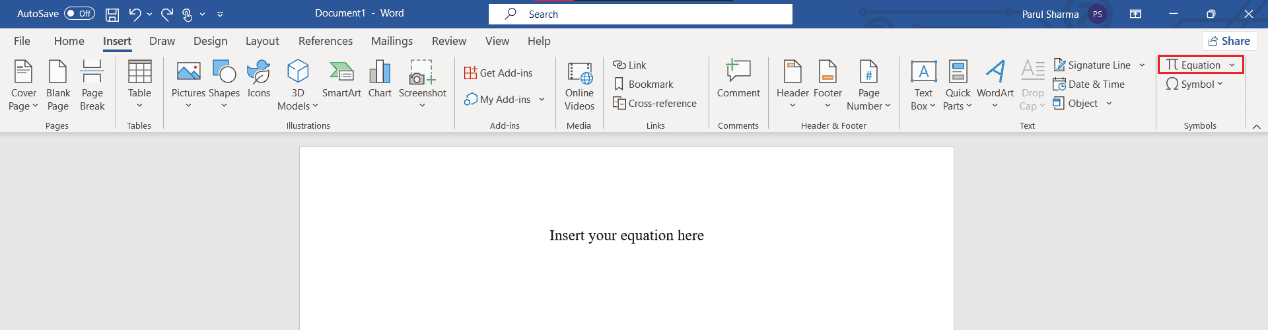3. Now, click on the Insert New Equation option.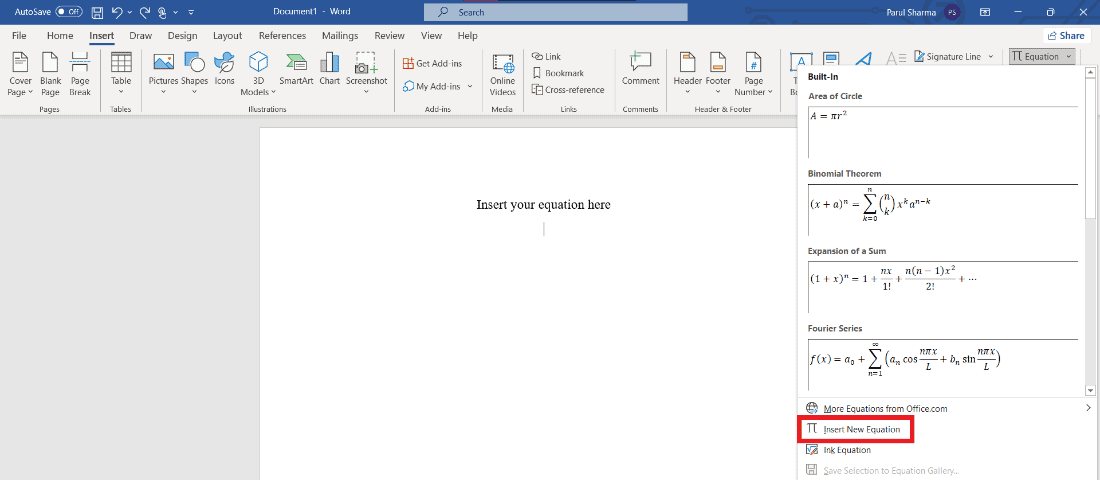4. In your working area, Type your Equation dialogue box will appear.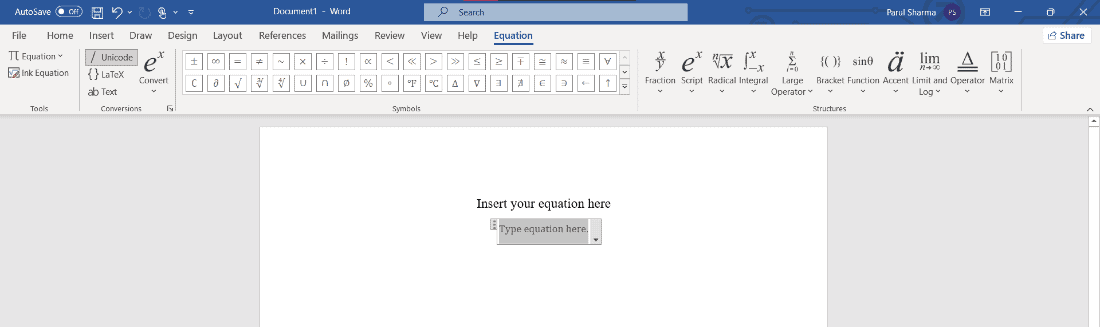You can further create the equation manually by using the symbols and structures from toolbar.

#### Method 3: Insert Equation using Keyboard Shortcuts

To insert equation in MS Word using keyboard shortcuts follow the below-mentioned steps:

1. Press Alt and = keys to display the Type equation dialogue box in the working area.

2. Type the symbol name using \followed by the symbol name. For example, type \theta.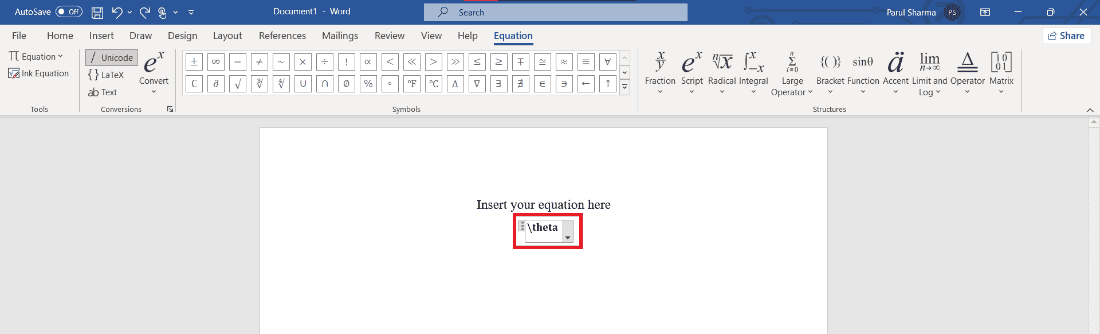3. Press spacebar key to convert it to the equation.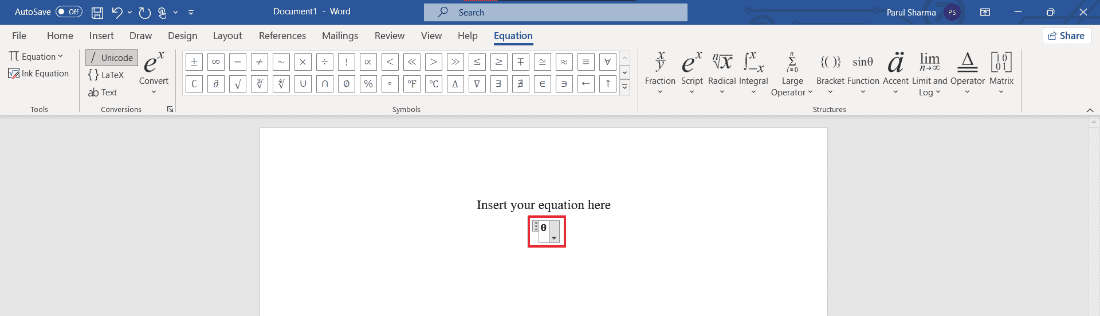This is how you can insert equation in Word using keyboard shortcuts.

### Most Common Microsoft Equation Editor Shortcuts

Here are the common equation editor shortcuts you may need for your Word documents:

 Shortcut To insert \infty ∞ \rightarrow → \leftarrow ← \downarrow ↓ \nearrow ↗ \searrow ↘ \nwarrow ↖ \swarrow ↙ \leftrightarrow ↔ \Rightarrow ⇒ \Uparrow ⇑ \partial 𝜕 \le ≤ \ll ≪ a\cdot b 𝑎⋅𝑏

Q1. What is the shortcut for insert Equation?

Ans. To use the keyboard shortcut for inserting an equation, press alt + = keys simultaneously.

Q2. Where is the equation tab in Word?

Ans. To find the Equation tab, go to Insert and click on Equation.

Q3. How do I get Microsoft equation editor

Ans. Microsoft equation editor is an in-built feature that spares you from the hassle of downloading thing separately. Thus, you can find it after clicking on Insert tab at the top.

Recommended

Microsoft equation editor is a life savior for many users. Although it is quite easy to understand, some people still consider it a tough nut to crack. We hope this article helped you in understanding how to insert equation in Word 2007 version and above. If so, let us know your reviews and suggestions in the comments down below.

Posted by

Elon Decker

Elon is a tech writer at TechCult. He's been writing how-to guides for about 6 years now and has covered many topics. He loves to cover topics related to Windows, Android, and the latest tricks and tips.